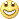# New metatrader 4 compatible indicators - page 2659

Happy trading in new week to all. My trailing stop EA only trailing when profit, and that's bad. Does anyone have trailing EA even not profit?160087

kjmchc1010:
Happy trading in new week to all. My trailing stop EA only trailing when profit, and that's bad. Does anyone have trailing EA even not profit?275

Here is this one. AutoPivotIndicator_ver5 does not need to be changed at all (it will work as is on a new builds of metatrader 4 too - just compile it)13

d_rsi.mq4Dear mladen. Faced with the problem after the upgrade terminal, namely code indicator using your idea with nolagma where it is called double iNoLag. The code is short. Help solve the problem pls

Files:
d_rsi.mq4 7 kb43

Hi

My request is to make this indicator compatible with new MT4 Build 610

"RSIOMA_v4.mq4"

Thanks

Files:
rsioma_v4.mq4 12 kb8

Got the beautiful surprise from Metaq. today!!!!! I want to thank them, %^&*&^%\$!!!!!!!

Dear Mladen, I will have to turn to you for help. I am no coder, so I have to ask you if you can re code two indicators to work with build 600 or 610, not sure if there is big difference in these two builds!!!!!!!!!!!

I have two MT4 platforms running simultaneously and all was fine until today. Today I did an update on one of them. The build is 600. The MT4 can load the two indicators that I need but the it works very slow. The other freshly DDL from FMXC with build 610 works fine but the indicators do not work. So I am like W.F!!!!!!!!!!!!!

I hope you can help

Files:
ssrc.mq4 7 kb160087

hektorian:

Got the beautiful surprise from Metaq. today!!!!! I want to thank them, %^&*&^%\$!!!!!!!

Dear Mladen, I will have to turn to you for help. I am no coder, so I have to ask you if you can re code two indicators to work with build 600 or 610, not sure if there is big difference in these two builds!!!!!!!!!!!

I have two MT4 platforms running simultaneously and all was fine until today. Today I did an update on one of them. The build is 600. The MT4 can load the two indicators that I need but the it works very slow. The other freshly DDL from FMXC with build 610 works fine but the indicators do not work. So I am like W.F!!!!!!!!!!!!!

I hope you can help

As far as I see SSRC should be this one : https://www.mql5.com/en/forum/183798/page17160087

keshav:
Hi

My request is to make this indicator compatible with new MT4 Build 610

"RSIOMA_v4.mq4"

Thanks

keshav

Here you go

Files:160087

xamil777:
d_rsi.mq4Dear mladen. Faced with the problem after the upgrade terminal, namely code indicator using your idea with nolagma where it is called double iNoLag. The code is short. Help solve the problem pls

xamil777

Replace non lag function with this :

#define Pi 3.14159265358979323846264338327950288

#define _length 0

#define _len 1

#define _weight 2

double nvalues[];

double nlalpha[];

double iNoLag( double &price[], int forvalue, int length, int r,int i)

{

int forValue = forvalue-1;

if (length<3) return(price[r]);

if (ArrayRange(nvalues,0)<(forValue+1) || nvalues[forValue][_length] != length)

{

double Cycle = 4.0;

double Coeff = 3.0*Pi;

int Phase = length-1;

if (ArrayRange(nvalues,0)<forValue+1) ArrayResize(nvalues,forValue+1);

nvalues[forValue][_length] = length;

nvalues[forValue][_len] = length*4 + Phase;

nvalues[forValue][_weight] = 0;

ArrayResize(nlalpha,nvalues[forValue][_len]);

for (int k=0; k<nvalues[forValue][_len]-1; k++)

{

if (k<=Phase-1)

double t = 1.0 * k/(Phase-1);

else t = 1.0 + (k-Phase+1)*(2.0*Cycle-1.0)/(Cycle*length-1.0);

double beta = MathCos(Pi*t);

double g = 1.0/(Coeff*t+1); if (t <= 0.5 ) g = 1;

nlalpha[k] = g * beta;

nvalues[forValue][_weight] += nlalpha[k];

}

}

if (nvalues[forValue][_weight]>0)

{

int len = nvalues[forValue][_len];

double sum = 0;

for (k=0; k < len-1; k++) sum += nlalpha[k]*price[r-k];

return( sum / nvalues[forValue][_weight]);

}

else return(0);

}

and replace ths line

nlag = iNoLag(nOsc,2,15,r,i);

with this

nlag = iNoLag(nOsc,1,15,r,i);

But there are few more errors in that code that should be corrected13

xamil777

Replace non lag function with this :

#define Pi 3.14159265358979323846264338327950288

#define _length 0

#define _len 1

#define _weight 2

double nvalues[];

double nlalpha[];

double iNoLag( double &price[], int forvalue, int length, int r,int i)

{

int forValue = forvalue-1;

if (length<3) return(price[r]);

if (ArrayRange(nvalues,0)<(forValue+1) || nvalues[forValue][_length] != length)

{

double Cycle = 4.0;

double Coeff = 3.0*Pi;

int Phase = length-1;

if (ArrayRange(nvalues,0)<forValue+1) ArrayResize(nvalues,forValue+1);

nvalues[forValue][_length] = length;

nvalues[forValue][_len] = length*4 + Phase;

nvalues[forValue][_weight] = 0;

ArrayResize(nlalpha,nvalues[forValue][_len]);

for (int k=0; k<nvalues[forValue][_len]-1; k++)

{

if (k<=Phase-1)

double t = 1.0 * k/(Phase-1);

else t = 1.0 + (k-Phase+1)*(2.0*Cycle-1.0)/(Cycle*length-1.0);

double beta = MathCos(Pi*t);

double g = 1.0/(Coeff*t+1); if (t <= 0.5 ) g = 1;

nlalpha[k] = g * beta;

nvalues[forValue][_weight] += nlalpha[k];

}

}

if (nvalues[forValue][_weight]>0)

{

int len = nvalues[forValue][_len];

double sum = 0;

for (k=0; k < len-1; k++) sum += nlalpha[k]*price[r-k];

return( sum / nvalues[forValue][_weight]);

}

else return(0);

}

and replace ths line

nlag = iNoLag(nOsc,2,15,r,i);

with this

nlag = iNoLag(nOsc,1,15,r,i);

But there are few more errors in that code that should be corrected

OMG thx mladen you best one